# MCAT Physical : Capacitors and Dielectrics

## Example Questions

← Previous 1

### Example Question #41 : Circuits

Capacitors with capacitances of 3 μF, 7 μF and 10 μF are wired in parallel. What is the capacitance of the circuit?

1. it cannot be determined without knowing the resistance of the circuit
2. it cannot be determined without knowing the time constant in the circuit
3. 20 μF
4. approximately 1.75 μF
5. none of these is correct

5

4

3

1

2

3

Explanation:

Response 3 is the correct choice.  Electrons will space themselves as far apart as possible, because of charge repulsion; therefore, in a parallel arrangement, they will jump onto each capacitor and “load it up.” The parallel capacitance is calculated by simply adding the individual values. The value would be about 1.75 μF if the three were connected in series, where the formula is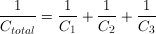(reciprocal of total equals sum of reciprocal of each). The time constant of a circuit is given by the formula τ = RC, and it relates to how fast a capacitor can charge to full capacitance.

### Example Question #41 : Circuits

A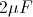and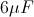capacitor are connected in series with a 12V battery. What is the maximum charge stored on thecapacitor?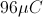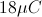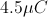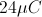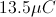Explanation:

First find the equivalent capacitance of the two capacitors by adding their inverses.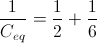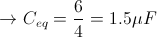Then, we can find the charge stored on this equivalent capacitor.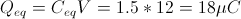For capacitors in series the charges on each must be equal, and also equal to the charge on the equivalent capacitor. The answer is.

### Example Question #43 : Circuits

Batteries and AC current are often used to charge a capacitor. A common example of capacitor use is in computer hard drives, where capacitors are charged in a specific pattern to code information. A simplified circuit with capacitors can be seen below. The capacitance of C1 is 0.5 μF and the capacitances of C2 and C3 are 1 μF each. A 10 V battery with an internal resistance of 1 Ω supplies the circuit.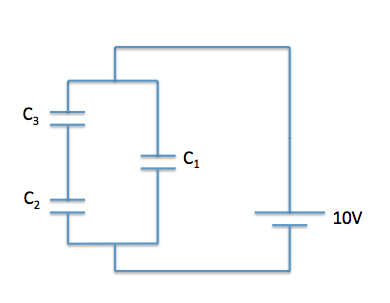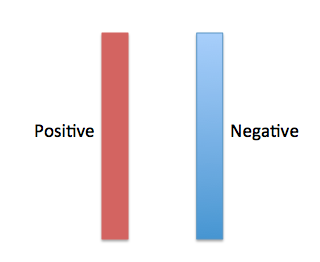How is the charge stored on the capacitor?

Unevenly distributed inside the capacitor

Evenly distributed inside the capacitor

Unevenly distributed on the capacitor surface

Evenly distributed on the capacitor surface

Evenly distributed on the capacitor surface

Explanation:

Charge is evenly distributed on the surface of the capacitor. If we think back to electric force, we know that positive charges repel other positive charges and negative charges repel other negative charges; thus, the charges are evenly distributed to minimize the force between them. We can see how this looks in diagrammatic form below.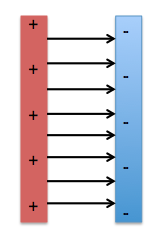### Example Question #44 : Circuits

Batteries and AC current are often used to charge a capacitor. A common example of capacitor use is in computer hard drives, where capacitors are charged in a specific pattern to code information. A simplified circuit with capacitors can be seen below. The capacitance of C1 is 0.5 μF and the capacitances of C2 and C3 are 1 μF each. A 10 V battery with an internal resistance of 1 Ω supplies the circuit.What is the equivalent capacitance of C2 and C3?

3μF

2μF

0.33μF

0.5μF

0.5μF

Explanation:

First, we need to determine how these capacitors are being added. We can see that they are being added in in series. Remember that capacitors in series are added as reciprocals: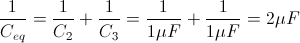Ceq = 0.5μF

### Example Question #45 : Circuits

Batteries and AC current are often used to charge a capacitor. A common example of capacitor use is in computer hard drives, where capacitors are charged in a specific pattern to code information. A simplified circuit with capacitors can be seen below. The capacitance of C1 is 0.5 μF and the capacitances of C2 and C3 are 1 μF each. A 10 V battery with an internal resistance of 1 Ω supplies the circuit.What is the equivalent capacitance of the circuit?

2μF

3μF

4μF

1μF

1μF

Explanation:

First, we need to determine how capacitors C2 and C3 are being added. We can see that they are being added in in series. Remember that capacitors in series are added as reciprocals.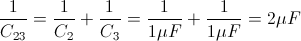C23 = 0.5μF

Next, we need to determine how we can find the Ceq by simplifying C23 and C1. We can see that Ceq and C1 are in parallel, thus we can directly add the individual capacitances.

Ceq = C23 + C1 = 0.5μF + 0.5μF = 1μF

### Example Question #46 : Circuits

Batteries and AC current are often used to charge a capacitor. A common example of capacitor use is in computer hard drives, where capacitors are charged in a specific pattern to code information. A simplified circuit with capacitors can be seen below. The capacitance of C1 is 0.5 μF and the capacitances of C2 and C3 are 1 μF each. A 10 V battery with an internal resistance of 1 Ω supplies the circuit.How long does it take to fully charge the capacitors of the circuit?

1 * 104s

1 * 103s

1 * 106

1 * 105s

1 * 106

Explanation:

In order to determine the time, we need to know the total charge stored on the capacitors. Remember that Q = CV, where Q is the total charge, C is the equivalent capacitance, and V is the voltage. We must first find the equivalent capacitance.

C2 and C3 are capacitors in series, while C1 is in parallel.C23 = 0.5μF

Ceq = C23 + C1 = 0.5μF + 0.5μF = 1μF

Now we can plug in the Ceq and battery voltage to find the charge.

Q = (1μF)(10V) = 10μC

Additionally, we need to know the current the battery can provide (the charge per unit time). Knowing both the total charge and current will allow us to calculate the time. We can use V = IR to determine the current.

I = V/R = 10V/1Ω = 10A = 10C/sec

We can equate charge and current to determine time.

10μC = 10 C/t

t = 10 C/10 μC = 1 * 106s or 11.6days

### Example Question #47 : Circuits

Batteries and AC current are often used to charge a capacitor. A common example of capacitor use is in computer hard drives, where capacitors are charged in a specific pattern to code information. A simplified circuit with capacitors can be seen below. The capacitance of C1 is 0.5 μF and the capacitances of C2 and C3 are 1 μF each. A 10 V battery with an internal resistance of 1 Ω supplies the circuit.Instead of air, assume that we insert a dielectric material with a dielectric constant k between the capacitor plates. How would the total capacitance of the circuit change?

Decrease

Increase

Remain the same

Increase

Explanation:

In this question, we are asked how the total charge stored on the surface of the capacitors would change if we inserted a dielectric between the parallel plates. As we can see in the equation for capacitance based on physical properties,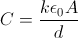, where k is the dielectric constant (k = 1 for air; k > 1 for all dielectric materials), ε0 is the constant of the permeability of free space, A is the area of the plates, and d is the distance between the plates.

If we insert a dielectric material, k > 1, the value of C increases, thus the overall capacitance of the circuit increases.

### Example Question #1 : Capacitors And Dielectrics

Which of the following statements about dielectrics is false?

A dielectric material increases voltage by decreasing stored charge

A dielectric material is non-conducting

A dielectric material increases capacitance by increasing stored charge

A dielectric material increases capacitance by decreasing voltage

A dielectric material increases voltage by decreasing stored charge

Explanation:

Dielectrics are put between two parallel plates of a capacitor to increase capacitance. This is done by holding voltage constant and increasing charge, or decreasing voltage and holding charge constant.

This can be understood using the equation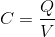. Either an increase in charge or a decrease in voltage will increase the capacitance.

### Example Question #49 : Circuits

Two capacitors are fully charged and connected in series in a circuit powered by a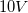battery. Find the charge stored.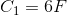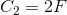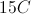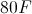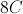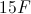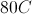Explanation:

First, we need to find the equivalent capacitance for the capacitors in series.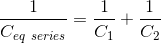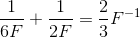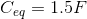Now we can find the charge using the equation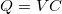.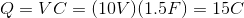### Example Question #81 : Electricity And Magnetism

Two parallel plate capacitors C1 and C2 are arranged in parallel within a 10V circuit. If C1 stores 2C of charge and C2 stores 5C of charge, each over 3 seconds, what is the total capacitance of the circuit?

0.2 F

0.7 F

2.4 F

1.8 F

0.7 F

Explanation:

The capacitance of any capacitor can be calculated with the formula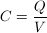Since the capacitors are arranged in parallel, the voltage drop across each will be equal, and in this case 10V. Adding capacitors in parallel is the same as adding resistors in series, where the total capacitance of the circuit is equal to the sum of all individual capacitors.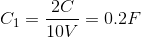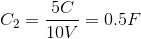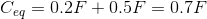← Previous 1